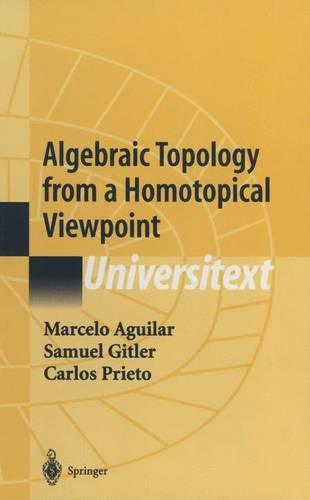•Algebraic Topology from a Homotopical Viewpoint - Universitext (Hardback)

(author), (author), (author)
£74.99
Hardback 479 Pages / Published: 13/06/2002
• We can order this

Usually dispatched within 3 weeks

The authors present introductory material in algebraic topology from a novel point of view in using a homotopy-theoretic approach. This carefully written book can be read by any student who knows some topology, providing a useful method to quickly learn this novel homotopy-theoretic point of view of algebraic topology.

Publisher: Springer-Verlag New York Inc.
ISBN: 9780387954509
Number of pages: 479
Weight: 985 g
Dimensions: 235 x 155 x 28 mm
Edition: 2002 ed.

MEDIA REVIEWS

From the reviews:

"This is a basic course on algebraic topology and ... it is excellently written and composed and can be strongly recommended to anybody wishing to learn the field. The reader can find many examples, calculations, and also a number of exercises. ... The book has two appendices, ... references consisting of 83 items and a long list of symbols. There are many remarks and comments making the orientation of the reader in the field of algebraic topology easier ... ." (EMS, June, 2004)

"The modus operandi of algebraic topology is to associate algebraic invariants, such as groups or rings, to a topological space in such a way that equivalent spaces exhibit isomorphic invariants; here, 'equivalent' may be chosen to fit the geometry of the problem. In this book, 'equivalent' means homotopy equivalent ... . For its clarity and directness, a welcome addition to advanced mathematics collections. Upper-division undergraduates through faculty." (J. McCleary, CHOICE, December, 2002)

"The purpose of this book is to introduce algebraic topology using the novel approach of homotopy theory ... . The basic concepts of homotopy theory ... are used to construct singular homology and cohomology, as well as K-theory. ... Throughout the text many other fundamental concepts are introduced ... ." (L'Enseignement Mathematique, Vol. 48 (3-4), 2002)

"The book of Aguilar, Gitler and Prieto gives an interesting new approach for a first course in algebraic topology. ... What is also very interesting is the fact that the book contains a detailed presentation of some deep results of algebraic topology not usually covered by a first book in algebraic topology ... . The text is carefully well written. ... the student in algebraic topology will find in the book a lot of interesting well-exposed material." (Yves Felix, Bulletin of the Belgian Mathematical Society, 2007)